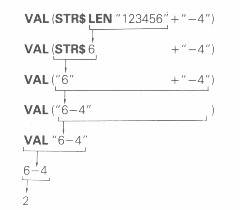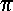Chapter 7 - Strings

One thing the ZX81 can do that pocket calculators cannot is deal with text.  Type

PRINT "HI THERE. I AM YOUR ZX81."       (" is shifted P.)

The chilling greeting inside the quotes is called a string (meaning a string of characters), & can contain any characters you like except the string quote,". (But you can use the so-called quote image, "" (shifted Q), & this will be printed as " by a PRINT statement.)

A common typing error with strings is to miss out one of the quotes - this will give you themarker.

If you are printing numbers out, you can use these strings to explain what the numbers mean. For instance, type

LET EGGS=61

& then

PRINT "THE PRICE OF EGGS IS ";EGGS;" NEW PENCE A DOZEN."

This statement displays three things (PRINT items), namely the string "THE PRICE OF EGGS IS ", the number 61 (the value of the variable EGGS), & then the string " NEW PENCE A DOZEN." In fact you can PRINT any number of items you like, & any mixture of strings & numbers (or expressions), note how the spaces in a string are just as much part of it as the letters. They are not ignored even at the end.

There are lots of things you can do with strings.

1. You can assign them to variables. However, the name of the variable must be special to show that its value is a string & not a number: it must be a single letter followed by \$ (shifted U). For instance, type

LET A\$="DOUBLE GLOUCESTER"

&

PRINT A\$

2. You can add them together. This is often called concatenation, meaning 'chaining together', & that is exactly what it does. Try

PRINT "GAMMO" + "N RASHERS"

You cannot subtract, multiply or divide strings, or raise them to powers.

3. You can apply some functions to strings to get numbers, & vice versa.

 LEN This is applied to a string, & the result is its length. For instance LEN "CHEESE" = 6. VAL This applied to a string, & the result is what that string gives when evaluated as an arithmetic expression. For instance (if A = 9), VAL "1/2+SQRA" = 3.5. If the string to which VAL is applied contains variables, then two rules must be obeyed. (i) If the VAL function is part of a larger expression, it must be the first item; e.g. 10 LET X = 7+VAL "Y" must be changed to 10 LET X = VAL "Y" +7. (ii) VAL can only appear in the first coordinate of a PRINT AT, PLOT or UNPLOT statement (see Chapter 17 and 18) e.g. 10 PLOT 5, VAL "X" must be changed to         10 LET Y = VAL "X"         15 PLOT 5, Y

STR\$ This is applied to a number, & the result is what would appear on the screen if the number were displayed by a PRINT statement. For instance STR\$ 3.5 = "3.5".

4. Just as for numbers, you can combine these to make string expressions, like

VAL (STR\$ LEN "123456"+"-4")

which is evaluated asSummary

Strings

Operation: + (for strings)

Functions: LEN, VAL, STR\$

Exercises

1. Type

LET A\$="2+2"

& then

PRINT A\$;" = ",VAL A\$

Try changing A\$ to more complicated things & doing the same, e.g.

LET A\$="ATN 1*4"

(The answer here should be.)

2. The string "" with no characters is called the empty or null string. It is only string whose length is 0. Remember that spaces are significant and an empty string is not the same as one containing spaces.

Do not confuse it with the quote image, "" (a single token, shifted Q). This is a special device to get over the fact that you cannot write an ordinary string quote in the middle of a string (why not?). When the quote image appears in a string that has its quotes at the end (for instance in the listing of a program), it shows up as two quote symbols, to distinguish it from the ordinary quote; but when it is displayed by a PRINT statement, it is as just one quote symbol.

Try

PRINT "X";"";"X",""""."""";"";""""

3. If you enjoy humiliating computers, type

PRINT "2+2 = ";2+1

Previous: Chapter 6    Next: Chapter 8